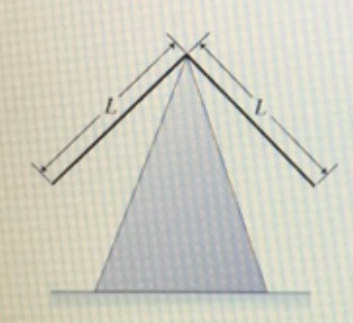# Problem: Two identical thin rods, each of mass m and length L are joined at right angles to form an L-shaped object. This object is balanced on top of sharp edge. If the object is displaced, it oscillates.Find w, the angular frequency of oscillation of the object.Your answer for the angular frequency may contain the given variables m and L as well as g.

###### FREE Expert Solution

The moment of inertia of the rod is expressed as:

$\overline{){{\mathbf{I}}}_{{\mathbf{1}}}{\mathbf{=}}\frac{\mathbf{1}}{\mathbf{3}}{\mathbf{m}}{{\mathbf{L}}}^{{\mathbf{2}}}}$

We can now express the angular frequency as:

79% (326 ratings)###### Problem Details

Two identical thin rods, each of mass m and length L are joined at right angles to form an L-shaped object. This object is balanced on top of sharp edge. If the object is displaced, it oscillates.Find w, the angular frequency of oscillation of the object.
Your answer for the angular frequency may contain the given variables m and L as well as g.